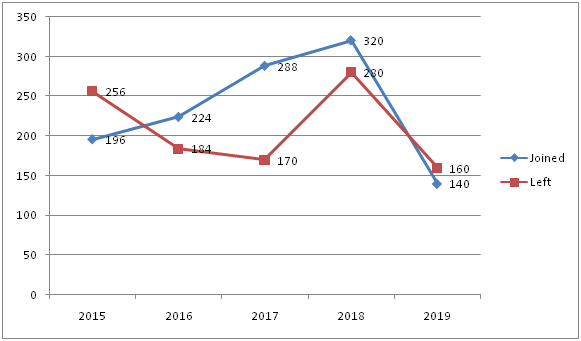# IBPS RRB PO Prelims Quantitative Aptitude Questions 2019 (Day-13)

Dear Aspirants, Our IBPS Guide team is providing new series of Quantitative Aptitude Questions for IBPS RRB PO Prelims 2019 so the aspirants can practice it on a daily basis. These questions are framed by our skilled experts after understanding your needs thoroughly. Aspirants can practice these new series questions daily to familiarize with the exact exam pattern and make your preparation effective.

Check here for IBPS RRB Prelims Mock Test 2019

### Click Here to Subscribe Crack High Level Puzzles & Seating Arrangement Questions PDF 2019 Plan

[WpProQuiz 6755]

Directions (1 – 5): What approximate value should come in the place of (?) in the following questions?

1) 33.85 * 15.12 + 27.23 * 86.95 – 42.35 * 32.46= ?

a) 1415

b) 1315

c) 1115

d) 1515

e) 1400

2) 362.85 * 484.21 ÷ 43.92% of 3298.98 = 32.98 * 899.75 ÷ 14.86 + ? – 2399.65

a) 521

b) 561

c) 481

d) 581

e) 541

3) 1819.89 ÷ 69.9 + 3/13.48 * 6.78/4.23 * 624.11 = 141.98 + ?

a) 136

b) 116

c) 156

d) 236

e) 216

4) 840.85 ÷ 57.78 * 11.65 + 61.122= ?–15.64% of 2499.85

a) 4395

b) 4595

c) 4295

d) 4495

e) 5295

5) 6125.24÷ 5/6 of (86.64 + 63.12)–6.84 * 3.99= ?

a) 51

b) 21

c) 11

d) 32

e) 55

Directions (6 – 10): Study the following information carefully and answer the questions given below.

Following line graph shows number of students who joined and left the school in the beginning of 5 years.

Total number of students in the school in 2014 = 9006) Find the number of students in the year 2018.

a) 1018

b) 1028

c) 1138

d) 1083

e) None of these

7) The strength of school increased/decreased from 2016 to 2019 by approximately what percentage?

a) 20% decreased

b) 24% increased

c) 4% decreased

d) 14% increased

e) None of these

8) Find the average number of students in the year 2017 to 2019.

a) 1018

b) 1028

c) 1016

d) 1081

e) None of these

9) The number of students in 2015 is what percentage of number of students in the year 2017?

a) 88%

b) 120%

c) 84%

d) 140%

e) None of these

10) Find the ratio of number of students in the year from which the maximum number of students left the school and the minimum number of students joined the school.

a) 1: 2

b) 519: 509

c) 2: 1

d) 509: 420

1. e) None of these

Direction (1-5) :

33.85 * 15.12 + 27.23 * 86.95 – 42.35 * 32.46 = ?

34 * 15 + 27 * 87 – 42 * 32 = ?

510 + 2349 –1344 = ?

1515 = ?

362.85 * 484.21 ÷ 43.92% of 3298.98 = 32.98 * 899.75 ÷ 14.86 + ? – 2399.65

363 * 484 ÷ 44% of 3300 = 33 * 900 ÷ 15 + ?– 2400

121 + 2400 –1980 = ?

541 = ?

1819.89 ÷ 69.9 + 3/13.48 * 6.78/4.23 * 624.11 = 141.98 + ?

1820 ÷ 70 + 3/13 * 7/4 * 624 = 142 + ?

26 + 252 = 142 + ?

136 = ?

840.85 ÷ 57.78 * 11.65 + 61.122= ? – 15.64% of 2499.85

841 ÷ 58 * 12 + 612= ? – 16% of 2500

174 + 3721 = ?–400

4295 = ?

6125.24 ÷ 5/6 of (86.64 + 63.12) – 6.84 * 3.99 = ?

6125 ÷ 5/6 of (87 + 63) – 7 * 4 = ?

6125 ÷ 125 – 28 = ?

21 = ?

Direction (6-10) :

The number of students in the year 2018 = 900 + (196 + 224 + 288 + 320)–(256 + 184 + 170 + 280)

= 1038

Strength of school in 2016 = 900 + 196 – 256 + 224 – 184 = 880

Strength of school in 2019 = 900 + (196 + 224 + 288 + 320 + 140) – (256 + 184 + 170 + 280 + 160)= 1018

Required percentage = ((1018 – 880)/1018) * 100 = 13.55% = 14% increased

Number of students in,

2015 = 900 + 196 – 256 = 840

2016 = 840 + 224 – 184 = 880

2017 = 880 + 288 – 170 = 998

2018 = 998 + 320 –280 = 1038

2019 = 1038 + 140 – 160 = 1018

Required average = (998 + 1038 + 1018)/3 = 1018

Number of students in,

2015 = 900 + 196 – 256 = 840

2016 = 840 + 224 – 184 = 880

2017 = 880 + 288 – 170 = 998

Required % = (840/998) * 100 = 84%

Number of students in,

2015 = 900 + 196 – 256 = 840

2016 = 840 + 224 – 184 = 880

2017 = 880 + 288 – 170 = 998

2018 = 998 + 320 – 280 = 1038

2019 = 1038 + 140 – 160 = 1018

Required ratio = 1038: 1018 = 519: 509